Next: MAP Estimation Up: CEM for Gaussian Mixture Previous: Bounding Vectors: The Gate

## Bounding Matrices: The Gate Covariances

Having shown the update equation for moving each gate's mean, we now turn our attention to the gate's covariance. Recall that each gating function was an unnormalized Gaussian as in Equation 7.14. Gaussians have no constraints on their mean which can be any real vector. Thus, it was possible to just bound the Q function with parabolas for the mean variable. However, Gaussians have constraints on their covariances which must remain symmetric and positive semi-definite. Thus, we can not use simple parabolic bounds trivially over all the parameters of the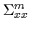matrices. Maximizing a parabola might return parameters forwhich don't maintain the above constraints. Thus, we explicitly bound the Q function with the logarithm of a single Gaussian density. Maximizing a Gaussian (as in maximum likelihood type calculations) is guaranteed to yield a symmetric positive definite matrix. Thus, we shall use it as a bound as in Equation 7.27. For compactness, we are merely writingas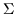.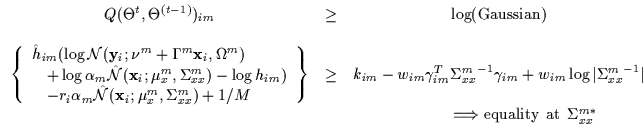(7.27)

Once again, we assume the data has been appropriately whitened with respect to the gate's previous parameters. Without generality, therefore, we can transform the data and assume that the previous value of the mean is 0 and the covariance is identity. We again solve for the parameters of the log-Gaussian bound using the three requirements (contact, tangentiality and tightness) and obtain the kim, him, wim as in Equation 7.28.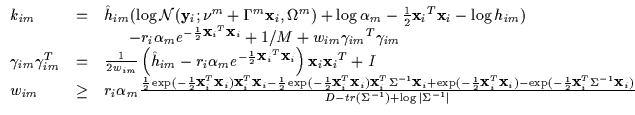(7.28)

As before, we would like an efficient way of solving for the minimal value of wim to get the tightest possible bound. In the definition, wim is lower bounded by a function of the covariance matrix which may contain very many parameters. We wish to find the maximum of this function (call it g) to find the minimum valid wim (which forms the tightest bound). Instead of exhaustively searching for the maximum of g by varying the many parameters of, we note a few simplifications. First, the covariance matrix can be written in terms of eigenvalues and eigenvectors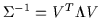. We also note that the denominator does not rely on the eigenvectors and can be written as in Equation 7.29.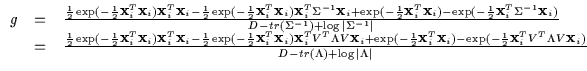(7.29)

The numerator of g can thus use V to further optimize gindependently of the denominator. As a consequence, one can vary the eigenvectors to select any eigenvalue in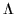by rotating the basis. Thus, the numerator only depend on the minimal and maximal values of the eigenvalue matrix (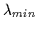and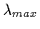). The denominator can then freely vary all other eigenvalues in between without affecting the numerator's range. Thus, we only need to search over different values ofandor two parameters. Using this and a further constraint which forces eitherorto be 1, we end up with a g function which is only a function of the magnitude of the data point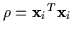and a single free scalar parameter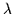as in Equation 7.30. This result was verified with numerical tests as well.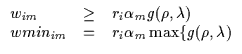(7.30)

Given a value of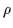from observed data, we need to find the best wminim by checking all the values of. In an offline process which requires a few seconds, we form a table of wminimfor eachusing Brent's method at each point. Thus, using this 1D lookup table, we can immediately compute the width of the bound given a data point. The values are shown in Figure 7.5 where we plot the max of the g function with respect to. Using this table, we can immediately compute the minimum wminim by looking up the corresponding value offor the current data point and subsequently compute the rest of the bound's parameters.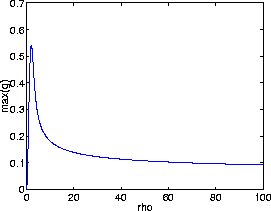Some examples of a log-Gaussian bound are shown in Figure 7.6 under different sub-components of the Qfunction. Each sub-component corresponds to a single data point as we vary one gate's uni-variate covariance. Many such parabolic bounds are computed (one for each data point and gate combination) and are summed to bound the Q function in its entirety.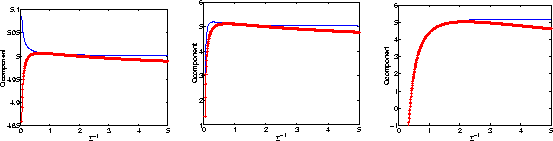To obtain a final answer for the update of the gate covarianceswe simply maximize the sum of log Gaussians which bounds the Q in its entirety. For M gates and N data points, we obtain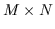bounds each with its own (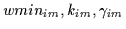). The update is in Equation 7.31.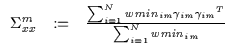(7.31)

This covariance is then unwhitened by inverting the transform used to move the data. This undoes the whitening operation which allowed us to transform data and consequently simplify intermediate calculations .Next: MAP Estimation Up: CEM for Gaussian Mixture Previous: Bounding Vectors: The Gate
Tony Jebara
1999-09-15